# Using the Quantity (qty) Field

When creating a project estimate, setting a quantity may be useful for calculating the price, calculating the amount of material(s) needed, and/or determining a subcontractor's fee.

Turn on Quantity

On a Project Task Group, click the  Set Qty button to toggle on the Quantity based measurement.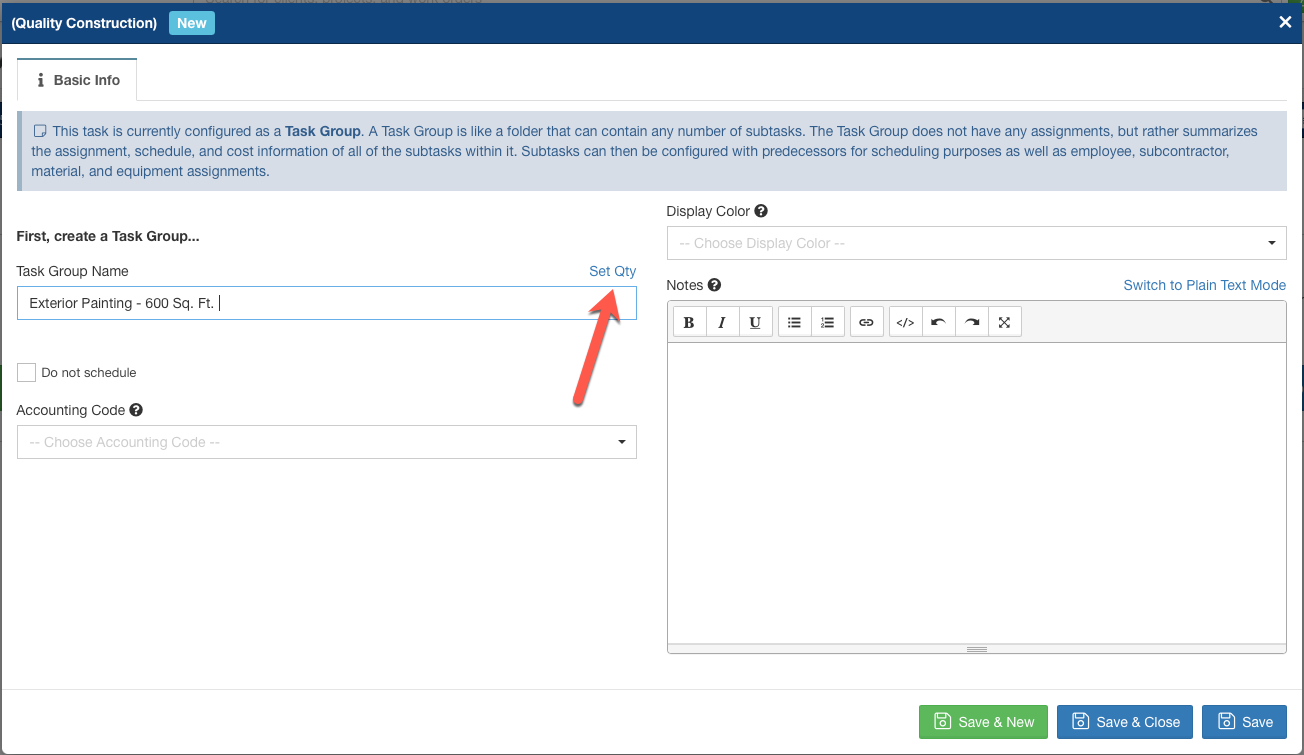Clicking Set Qty will toggle on Quantity based measurements. We can then use this Quantity to calculate a price, measure material cost, or determine Subcontractor fees.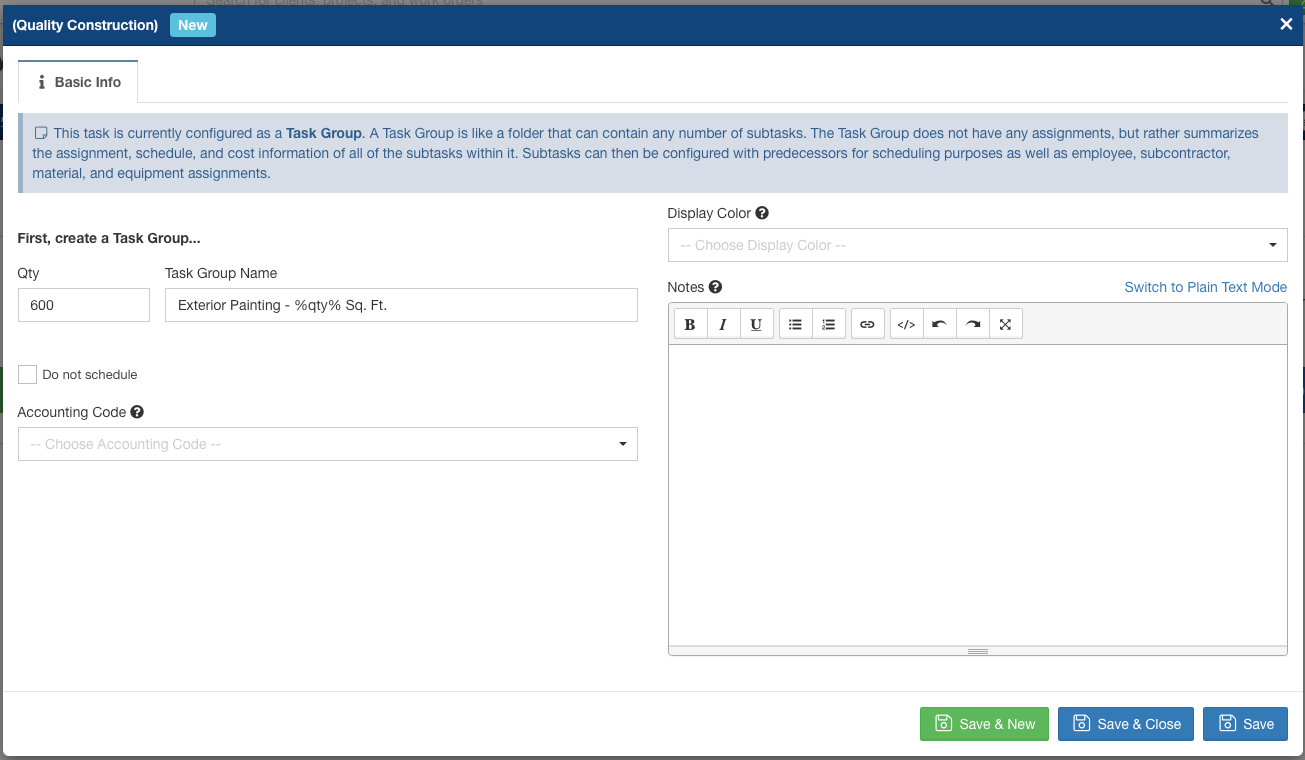Pro Tip: In the Task Group Name, use the tag %qty% to act as a placeholder variable. It will be replaced by whatever number is in the Qty field. In the above case, this Task Group Name will be "Exterior Painting - 600 Sq. Ft." when saved. It is helpful to note here what unit of measurement we're using for our Quantity. Square Feet, Linear Feet, United Inches, Per Unit, are all common quantity based measurements.

Setting a Price using Quantity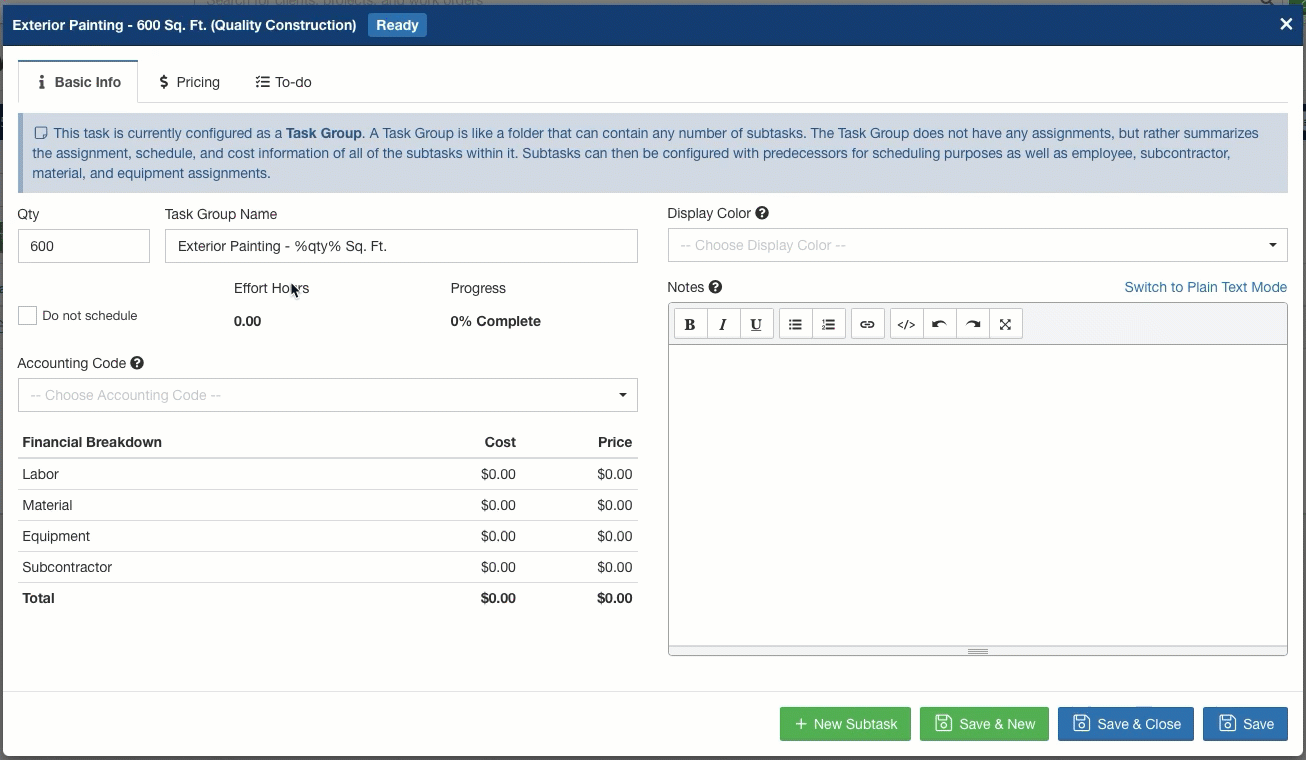Now clicking into the Pricing tab from this Task, we can set the Price Per Quantity. In the above example, the Quantity on this task is 600 (Sq. Ft.) multiplied by \$4.15, for a total price of \$2,490.

Calculating Cost Using Quantity

You can also link the Task Quantity to automatically calculate the amount of materials needed for that particular task.

First, set the Quantity on the Task Group as before: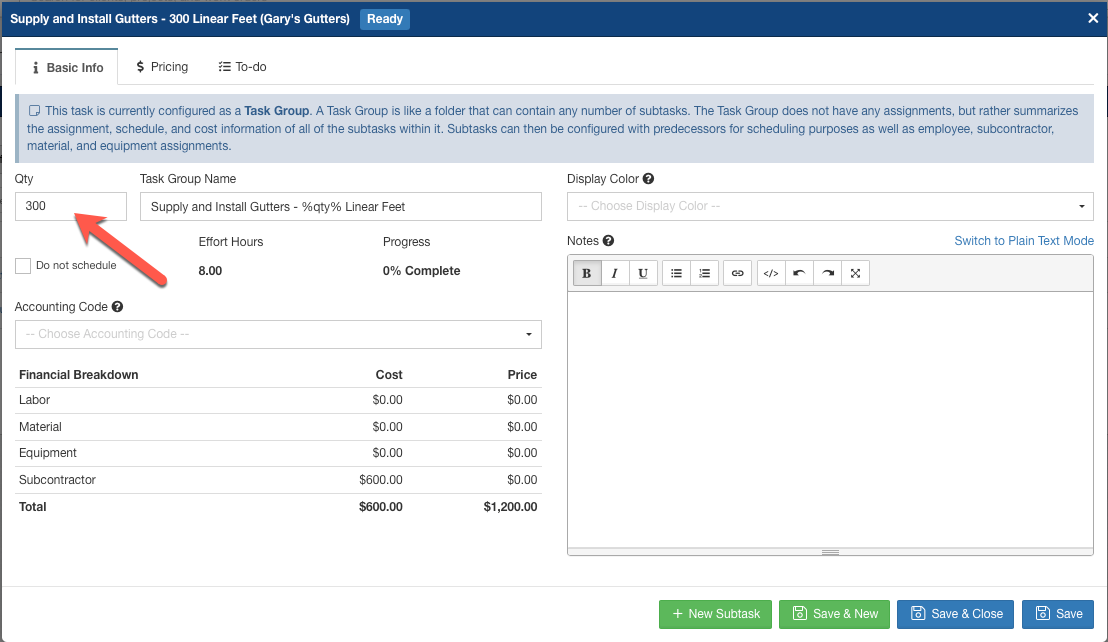In this project, we're Installing Gutters. Using the Quantity Field, we're creating this project for 300 Linear Feet (Note use of the %qty% tag, which will display 300 in this case).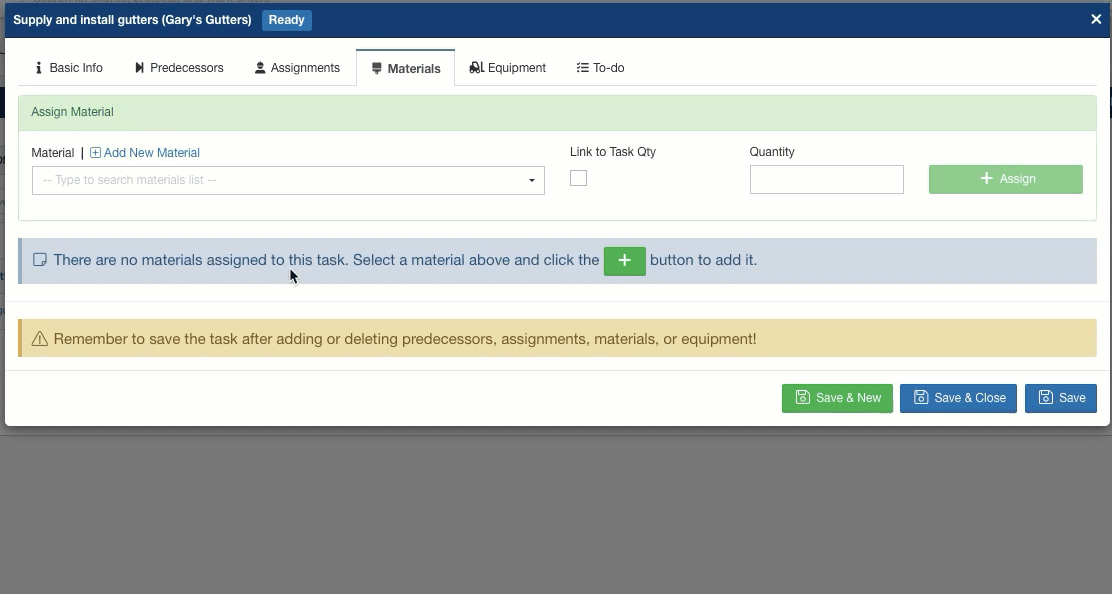We'll account for the Gutter Material, at \$1.50 per Lin. Foot. Since our cost basis is measured the same as our price basis, we'll link the Material amount to the Task Quantity of 300 Linear Feet.

Using Quantity to Calculate Subcontractor Costs

First, we must have a Subcontractor Flat Rate created. Head to Admin > Configure to find the Subcontractor Flat Rates widget. Add a new Flat Rate, and input the Cost per Unit. In this example, we're paying \$2 per Unit, or Linear Foot, for Gutter Installation: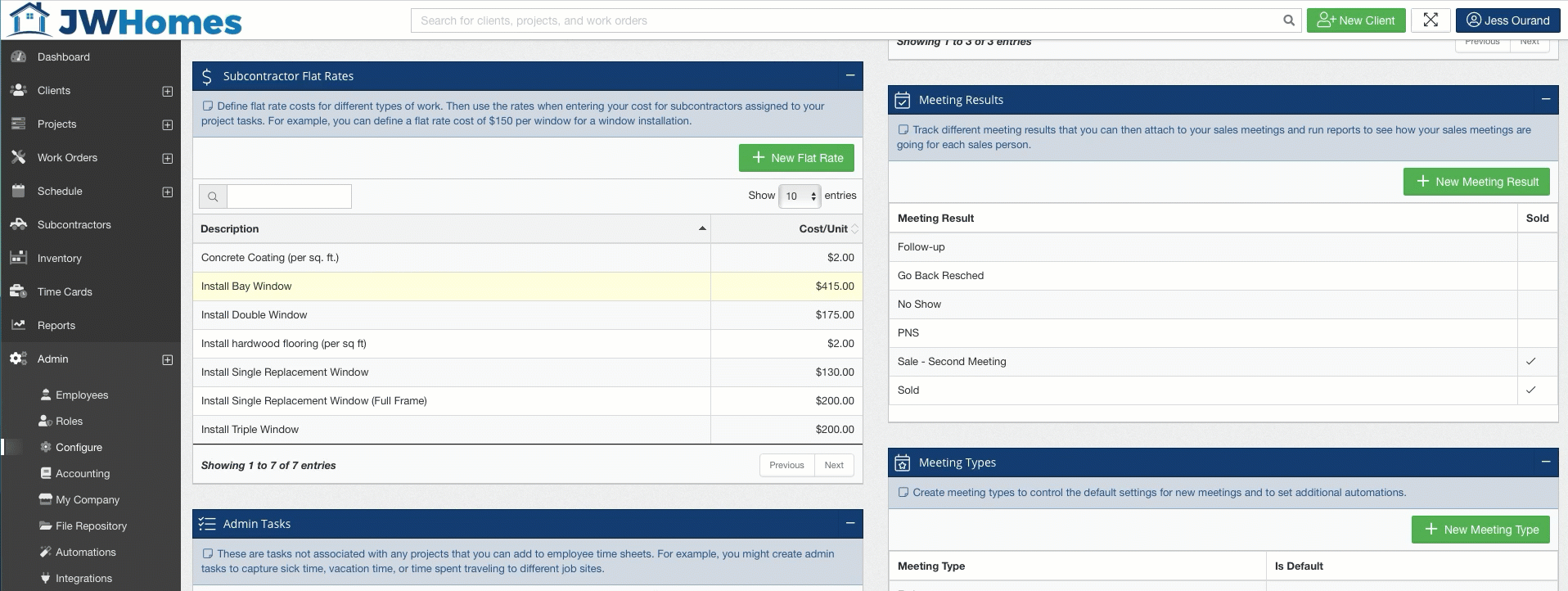We're creating a Subcontractor Flat Rate above. For installing Gutters, we'll pay \$2.00/ Lin. Foot.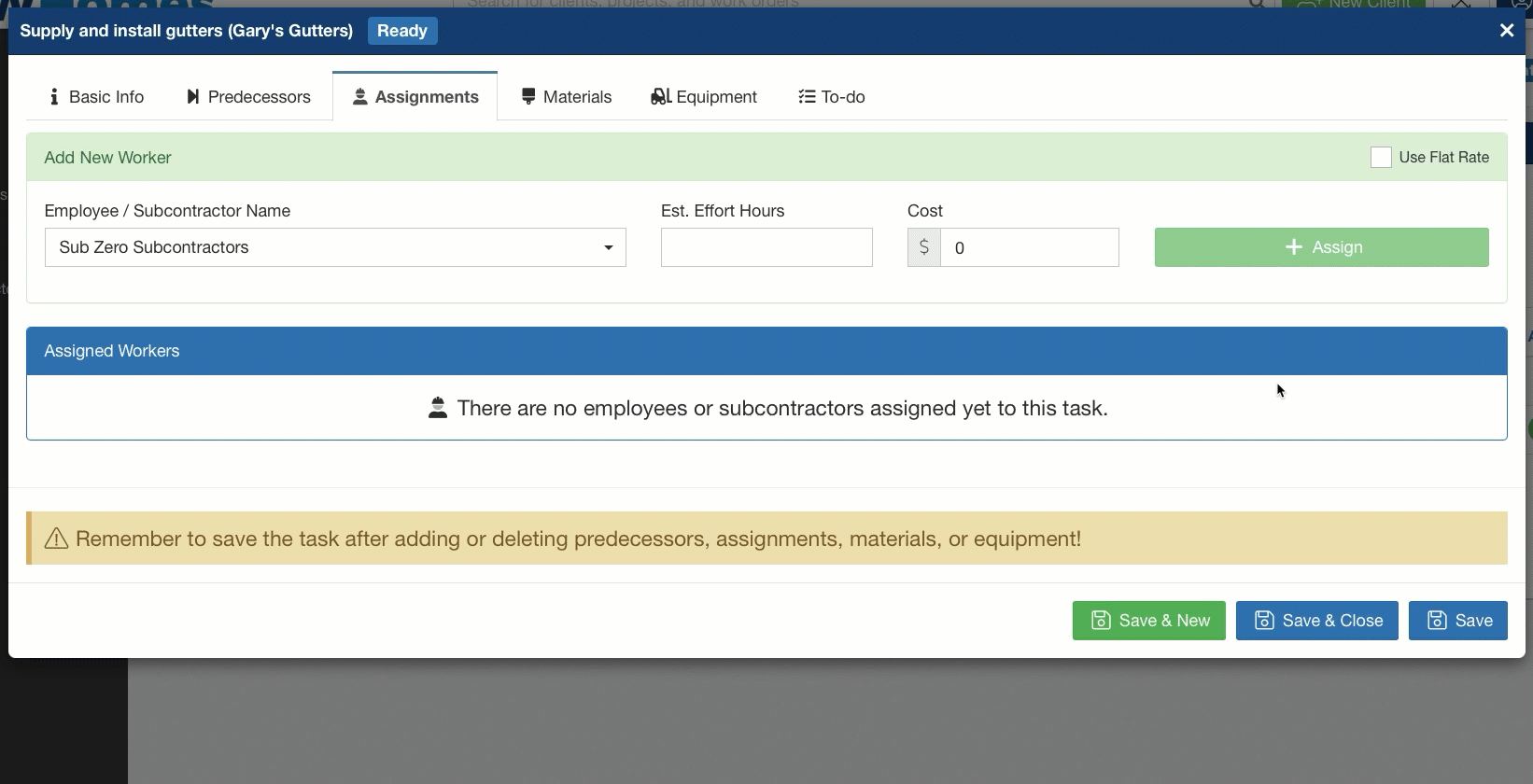Now when we assign a Subcontractor to this Project Subtask, we'll define the cost basis for the Subcontractor. We can manually set a cost, or we can use the Flat Rate we previously defined.